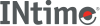﻿ scalb, scalbn (IEEE)INtime SDK Help
scalb, scalbn (IEEE)

The scalb function computes `x * rn`, where `r` is the radix of the machine's floating-point arithmetic. When `r` is 2, scalb shall be equivalent to ldexp. The value of `r` is FLT_RADIX which is defined in <float.h>. The scalbn function computes `x * FLT_RADIXn` efficiently.

```#include <math.h>

double scalb (double x, double n);

double scalbn (double x, int n);
```

#### Return Values

Upon successful completion, these functions shall return `x * FLT_RADIXn`.

If the result would cause overflow, a range error shall occur and these functions shall return +/-HUGE_VAL, (according to the sign of `x`).

#### Requirements

Versions Defined in Include Link to
INtime 3.0 intime/rt/include/math.h math.h clib.lib